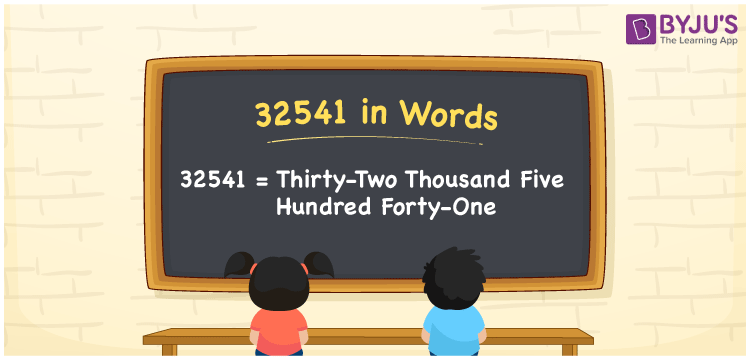# 32541 in Words

We can write 32541 in words as Thirty-two thousand five hundred forty-one. That means the word form or number name of 32541 is Thirty-two thousand five hundred forty-one rupees. We generally use the word form of 32541 to express, spell or communicate the same to others. Also, we can say that 32541 is a cardinal number since it denotes a specific amount.

 32541 in words Thirty-two thousand five hundred forty-one Thirty-two thousand five hundred forty-one in Numbers 32541

## 32541 in English Words

Generally, we use the English alphabet to express numbers in words. So, we spell 32541 in English words as “Thirty-two thousand five hundred forty-one”.## How to Write 32541 in Words?

In this section, you will learn how to convert the number 32541 into words with the help of a place value chart, which is given below.

 Ten thousands Thousands Hundreds Tens Ones 3 2 5 4 1

Here, ones = 1, tens = 4, hundreds = 5, thousands = 2, ten thousands = 3

These numbers can be written as:

3 × Ten thousand + 2 × Thousand + 5 × Hundred + 4 × Ten + 1 × One

= 3 × 10000 + 2 × 1000 + 5 × 100 + 4 × 10 + 1 × 1

= 30000 + 2000 + 500 + 40 + 1

= Thirty Thousand + Two thousand + Five hundred + Forty + One

= Thirty-two thousand five hundred forty-one

Thus, 32541 in words = Thirty-two thousand five hundred forty-one.

As we know, 32541 is a natural number that is the successor of 32540 and the predecessor of 32542.

32541 in words – Thirty-two thousand five hundred forty-one

Is 32541 an even number? – No

Is 32541 an odd number? – Yes

Is 32541 a prime number? – No

Is 32541 a composite number? – Yes

Is 32541 a perfect square number? – No

Is 32541 a perfect cube number? – No

## Frequently Asked Questions on 32541 in Words

Q1

### How do you write 32541 in words?

We can write 32541 in words as given below: 32541 = Thirty-two thousand five hundred forty-one
Q2

### How to write an amount of Rs. 32541 in words on a cheque?

On a cheque, we can write an amount of Rs. 32541 in words as “Thirty-two thousand five hundred forty-one rupees only”.
Q3

### What is the number name of 32541?

The number name of 32541 is Thirty-two thousand five hundred forty-one.